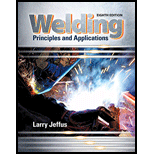# Referring to the graph in Figure 3-9, what would the voltage be for the CC power supply at 110 amps? What would the watts be?### Welding: Principles and Applicatio...

8th Edition
Larry Jeffus
Publisher: Cengage Learning
ISBN: 9781305494695

#### Solutions

Chapter
Section### Welding: Principles and Applicatio...

8th Edition
Larry Jeffus
Publisher: Cengage Learning
ISBN: 9781305494695
Chapter 3, Problem 13R
Textbook Problem
480 views

## Referring to the graph in Figure 3-9, what would the voltage be for the CC power supply at 110 amps? What would the watts be?

To determine

The voltage for the CC power supply.

The CC power supply in watts.

### Explanation of Solution

Given Information:

The electric current is 110amps.

Writing the expression for the power,

W=VI...... (I)

Here, the power is W, the voltages is V and the electric current is I.

Calculation:

Refer the Figure 3-10, "Constant voltage and Constant current" to obtain the value of voltage is 28.88volts corresponding to 110amps.

Substitute 110amps for I and 28.88volts for V in Equation (I).

W=(110amps)(28

### Still sussing out bartleby?

Check out a sample textbook solution.

See a sample solution

#### The Solution to Your Study Problems

Bartleby provides explanations to thousands of textbook problems written by our experts, many with advanced degrees!

Get Started

Find more solutions based on key concepts
Solve the following set of equations using MATLAB. [7.111.230001.231.990.760000.760.8510.0910000.0912.312.22000...

Engineering Fundamentals: An Introduction to Engineering (MindTap Course List)

Explain the issues surrounding companies using customer data.

Enhanced Discovering Computers 2017 (Shelly Cashman Series) (MindTap Course List)

Briefly describe the differences between a credit, charge, debit and smart card.

Principles of Information Systems (MindTap Course List)

Define encapsulation, and explain how it is used in object-oriented analysis.

Systems Analysis and Design (Shelly Cashman Series) (MindTap Course List)

If your motherboard supports ECC DDR3 memory, can you substitute non-ECC DDR3 memory?

A+ Guide to Hardware (Standalone Book) (MindTap Course List)